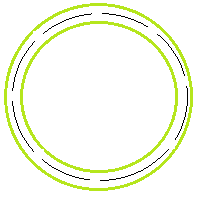# What is the electric potential on this conducting shell?

fangrz

## Homework Statement

If you have a conducting shell with charge Q surrounding a conducting sphere of charge negative - Q, what is the potential at the outside of the conducting shell? What about the inside?

V=(kQenclosed)/r

## The Attempt at a Solution

I don't know if the above equation holds true in this case. Are these assumptions correct:
1. On the outside of the conducting shell, potential is 0?
2. On the inside, the potential is kQ/R where R is the radius of the conducting shell?

First find the charge distribution on the inner surface and outer surface of both shells.
to solve such problems, keep these points in mind.
1) the whole charge of innermost shell comes to its outer surface unless a charge is kept inside it.
2)if you draw gausian surface inside the next shell, then the sum of all charges inside the surface has to be zero.
example, if q1 charge is on outer surface of innermost shell and q2 charge is on inner surface of next shell, these are the charges enclosed by the gausian surface and you can say q1+q2=0.
So equal and opposite charges appear on opposite faces.
3)if any shell is earthed, then its potential is zero.

•fangrz
fangrz
Oh, okay. So if I have a conducting shell of charge -Q around a conducting sphere of charge +Q, then the -Q charge will be pulled to the inside of the conducting shell.

1. So, on the outside of the conducting shell, do I have a potential of 0?
2. On the inside of the shell, do I have a potential of kQ/r?

Thanks!

fangrz
Because technically, the "q enclosed" for number 2 (for the inside of the shell) is still only the charged sphere inside right?

fangrz
First find the charge distribution on the inner surface and outer surface of both shells.
to solve such problems, keep these points in mind.
1) the whole charge of innermost shell comes to its outer surface unless a charge is kept inside it.
2)if you draw gausian surface inside the next shell, then the sum of all charges inside the surface has to be zero.
example, if q1 charge is on outer surface of innermost shell and q2 charge is on inner surface of next shell, these are the charges enclosed by the gausian surface and you can say q1+q2=0.
So equal and opposite charges appear on opposite faces.
3)if any shell is earthed, then its potential is zero.
And Thank you for your help! I just have those 2 issues that I listed above.

Start from inner shell. Draw a spherical gaussian surface inside its material like this. The black broken line represents the surface.So inside that surface the charge enclosed should be zero, since the field inside is zero.
So all of the charge has only one option., and that is to move to the outer surface.
Now equal and opposite charges will be induced. so -q charge is induce on the next shell's inner surface an let Q charge appear on its outer surface.But the net charge has to be -q. So Q=0.
Now can you give the charge distribution.

For potential on outside shell:
Potential due to inner surface of inner shell=0
Potential due to outer surface of inner shell=?
Potential due to inner surface of outer shell=?
Potential due to outer surface=0

Hint: Potential at any inside point of a shell of radius R, charge Q=##\frac{kQ}{R}## (independent of its position from center)
Potential at surface of a shell of radius R, charge Q=##\frac{kQ}{R}##
Potential at outside point =##\frac{kQ}{d}## where d is the distance from center of shell.

Homework Helper
Gold Member
You should always be clear about where you are taking the potential to be zero. Generally, for problems like this where the conductors are of finite size, the potential is chosen to be zero at infinity.

So, start at infinity where you know V = 0 and move from there to the outer surface of the outer conducting shell. (Think about the electric field in this region).

Then move from the outer surface of the outer shell to the inner surface of the outer shell. (Again think about the electric field in this region.)

From there you can move to points between the outer shell and the inner sphere.